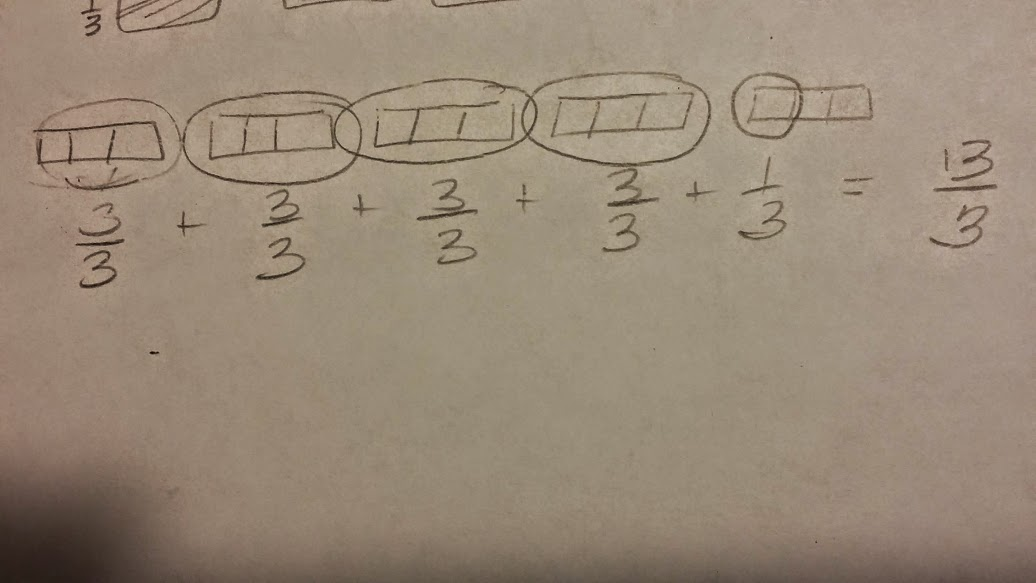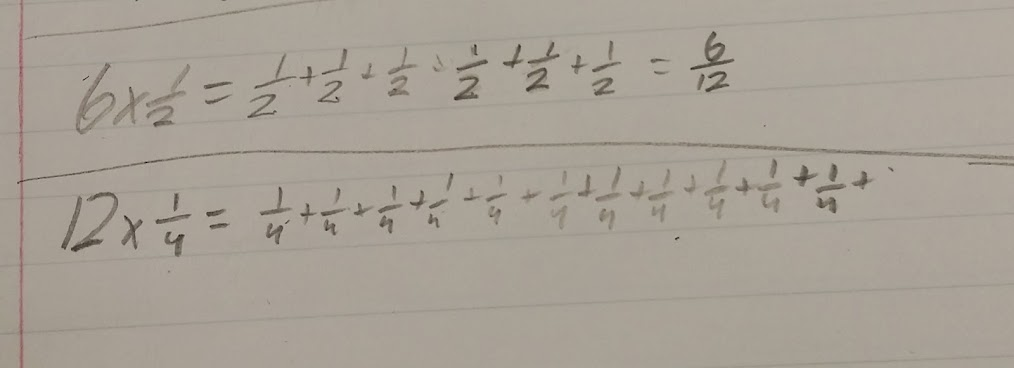# Multiplying Whole Number with a Fraction

18 teachers like this lesson
Print Lesson

## Objective

SWBAT prove that a fraction a/b is a multiple of unit fraction 1/b

#### Big Idea

In this lesson, students will multiply a whole number with a unit fraction through modeling in order to build conceptual understanding.

## Warm Up

5 minutes

For this warm up I use the strategy of white-boarding and ask students to find equivalent fractions by using common numerators or common denominators. This is a review skill, so as students are working to find equivalent fractions, I quickly circulate around the room noting which students are struggling and which students seem to have this skill mastered.

## Concept Development

50 minutes

In this lesson students work on fraction 4.NF.4.C - Understand a fraction a/b as a multiple of 1/b. For example, use a visual fraction model to represent 5/4 as the product 5 × (1/4), recording the conclusion by the equation 5/4 = 5 × (1/4).

My students have had prior experiences and lessons with adding unit fractions and fractions with like denominators which is a critical understanding before students will be successful with this lesson. In order for students to make connection between representing a fraction like 5/4 as the product of 5 x (1/4) students must be successful in knowing that 1/4 + 1/4 + 1/4 + 1/4 + 1/4 = 5/4 first.

To begin this lesson, I first display the fraction 13/3 and ask students what an improper fraction is.  Students respond by saying, "the numerator is larger than the denominator."  When students respond with this,  I guide them into also stating that this means the number (fraction) is greater than 1.

Again, my students have had some exposure to improper fractions through an everyday math computer program they participate in as well as by asking me.  I have found that many of my students have a natural curiosity about fractions and ask me many questions before concepts are presented.

I then instruct through direct instruction how to model the improper fraction 13/3 using an area model. As I model, students copy the model into their math notebooks.  I tell my students to imagine 13 dogs and I draw a dog face on the board.  Then I tell them that each dog eats 1/3 of a can of dog food each night. I am wondering how many total cans of food the dogs eat in one night. Some of my students have devised computation methods to create a mixed number from an improper fraction, but I insist that they know and be able to use a fraction model, especially since 4.NF.4.C lists a visual fraction model as an example in understanding a fraction a/b as a multiple of 1/b.

With 13/3 most students copied my example. For this next problem I ask students to attempt to model it first, before I show my model.Students then model 21/5 in their notebooks. Scaffolding the approach by not simply creating a model for students to copy into their notebooks is important in order for students to wrestle some with this concept.

Next, I refer back to the 13/3 fraction and the dogs. I ask student to think about this expression and the dog example:

13 x 1/3

I then listen to their observations. Students make connections that since each dog is having some of the can of dog food, then they can multiply to find the total cans.  Some students respond to that comment by saying, "No you would add 1/3 + 1/3 + 1/3 ... etc thirteen times. This is a great and important conversation to have with students as they work to expand their knowledge of multiplication as repeated addition.  Most students realize that 13 x 1/3 results in the same fraction as 1/3 + 1/3 + 1/3 + 1/3 + 1/3 + 1/3 + 1/3 + 1/3 + 1/3 + 1/3 + 1/3 + 1/3 + 1/3.

Next I display the multiplying a number by a fraction page. multiplying-a-number-by-a-fraction.pdf

I ask students to complete 1a, 1b, and 1c on their own first.  Once they see their learning partner is finished, they then turn and partner share their ideas and products with their partner.  Together, students then write at least three sentences to answer the questions in the middle of the page about the product of a number multiplied by a fraction less than 1.

You can see in this student's work sample, this student is still working to solidify the idea of multiplication  of a fraction by a whole number as repeated addition. This student is very comfortable with repeated addition.  Just below this photo, you can see and hear my conversation with this student about her work.## Student Debrief

10 minutes

For this lesson debrief, student partners share their ideas about multiplying a number by a fraction and what they notice happens to the product of that number from the worksheet.  Most of my students could see and identify that the product is less than the whole number, but a few students still need more time with this concept before they are able to make justifications about this idea.  I chose to end my lesson this this wrap up because this gives students opportunities to practice making conjectures and justifications as called for in Math Practice Standard 3.  Any opportunity students have to practice this skill only makes them stronger.